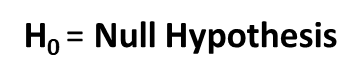# Null-Hypothesis: Epidemiology – a Beginner’s GuideResearch begins with ‘Null Hypothesis’ as the baseline.
Data is then gathered and analyzed to determine if there is enough evidence to reject the Null hypothesis in favor of an alternative hypothesis.
The evidence to reject or accept the Null hypothesis lies in calculating the p-value.

Null – Hypothesis (represented by H0) is an assumption that:

1. If two or more groups are being compared: there is no ACTUAL difference between these groups. Any difference found is merely by chance and is due to biological/sampling variability.
E.g. the mean height of male students in a school is 170 cm and of female students is 160 cm. H0 states that both male and female students have similar heights. The observed difference is only due to chance variation. It may not be seen the next time their heights are measured

2. If a relationship between variables is under investigation: Null-Hypothesis states that is NO Association between the two variables
E.g. there is no correlation between income and education level

3. If the effect of a drug or intervention is being investigated: Null-Hypothesis states that there is no actual effect. Any change observed is due to chance.
E.g. the mean systolic BP of a group on drug is 124 mm of Hg and that of placebo is 140 mm of Hg. H0 says that the drug actually has no effect on BP. The lower BP in drug group is just by chance due to biological variability.

A general form of the null hypothesis (H0): There is no significant difference or effect (between groups or variables) or no relationship, UNLESS proved otherwise!

p-value is the probability (b/w 0 to 1) of Null - hypothesis being TRUE

Hence if p-value is high, it means that there is the Null-Hypothesis is true and there is
• NO difference between the groups or
• No effect of the treatment under investigation or
• No correlation between two variables being tested

A LOW p-value implies a low probability of Null-Hypothesis being true.
Low p-value proves it otherwise and leads to REJECTION of Null-Hypotheses
i.e.
• The groups are actually different from each other
• The treatment under investigation HAS an effect
• The variables under study are correlated

What is the cut-off for high and low p-value?
The convention cut-off of the p-value is 0.05.

If p ≥ 0.05, Null-Hypothesis is true and accepted. There is no difference between the groups/no effect of intervention/no association between variables
If p < 0.05, Null Hypothesis is rejected and any observed difference or association is taken to be a REAL one.

When the Null-Hypothesis is rejected, it means that the alternative hypothesis (H1) is accepted. H1 says that the difference between the groups is real, the intervention HAS an effect or there IS an association between the variablesReferences:
1. Park K. Health information and basic medical statistics. In: Park’s Textbook of Preventive and Social